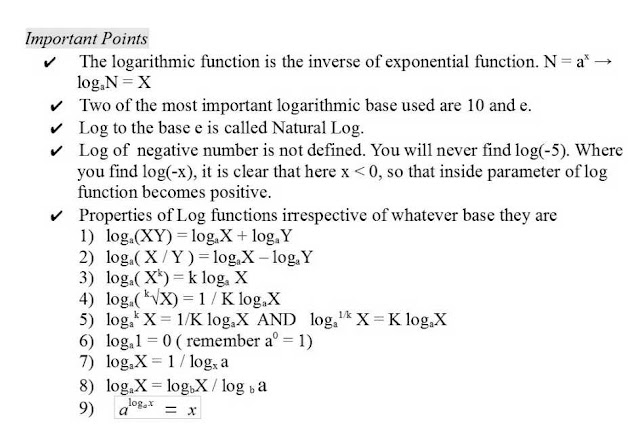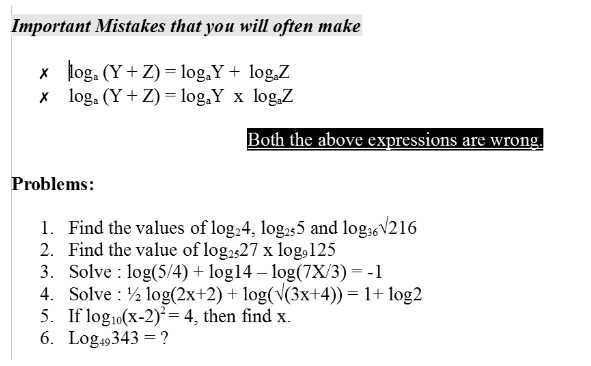### LOGARITHMS

Logarithm and logarithmic based functions and questions can be framed in every section of mathematics from algebra to calculus and even geometry. But the most confusing and difficulty faced is in the calculus and differentiation and continuity when the question is combined with multiple concepts in the scene. It becomes difficult to handle the question if you do not know in detail about how to move around with logarithms.

Most of you must have got confused with logarithms raised to powers and powers raised to log functions. Yes, it will become more confusing if such things appear in calculus, at that point of time you will have to also keep in mind the continuity, domain, range and base. Yes, another important thing is 'Base'.
Many of you might not even know how the base is changed. Remember if you are not used to such simple simple things, you will get stuck in the middle of any question and to your surprise even you might get stuck at the last stage of your solution because you are helpless to change base, as you dont know how to do it !!

So why not just spend a few minutes, to understand how actually you can mover around playing with indices, properties of log functions and changing bases and raising powers to numbers and even bases of log functions.

Important Points
The logarithmic function is the inverse of exponential function. N = ax → logaN = X
Two of the most important logarithmic base used are 10 and e.
Log to the base e is called Natural Log.
Log of negative number is not defined. You will never find log(-5). Where you find log(-x), it is clear that here x < 0, so that inside parameter of log function becomes positive.

Click on the question to enlarge__________________________________